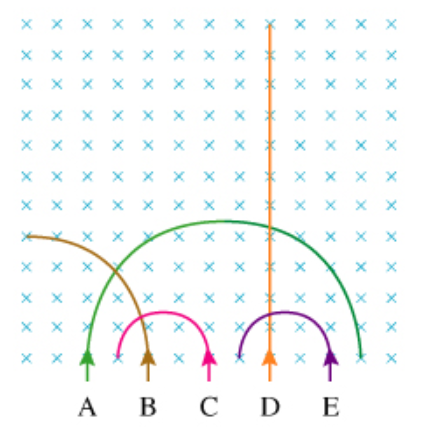# Problem: Rank the particles on the basis of their speed.Rank from largest to smallest.

###### FREE Expert Solution

Magnetic force:

Centripetal force:

$\overline{){{\mathbf{F}}}_{{\mathbf{c}}}{\mathbf{=}}{\mathbf{m}}\frac{{\mathbf{v}}^{\mathbf{2}}}{\mathbf{r}}}$

The magnetic force provides the centripetal force. Therefore,

F = Fc

qvB = mv2/r

r = mv2/qvB = mv/qB

81% (26 ratings)###### Problem DetailsRank the particles on the basis of their speed.

Rank from largest to smallest.